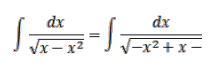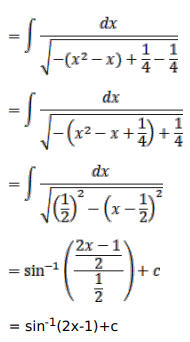# Mark against the correct answer in each of the following:

Question:

Mark $(\sqrt{ })$ against the correct answer in each of the following:

$\int \frac{d x}{\sqrt{x-x^{2}}}=?$.

A. $\sin ^{-1}(x-1)+C$

B. $\sin ^{-1}(x+1)+C$

C. $\sin ^{-1}(2 x-1)+C$

D. none of these

Solution: